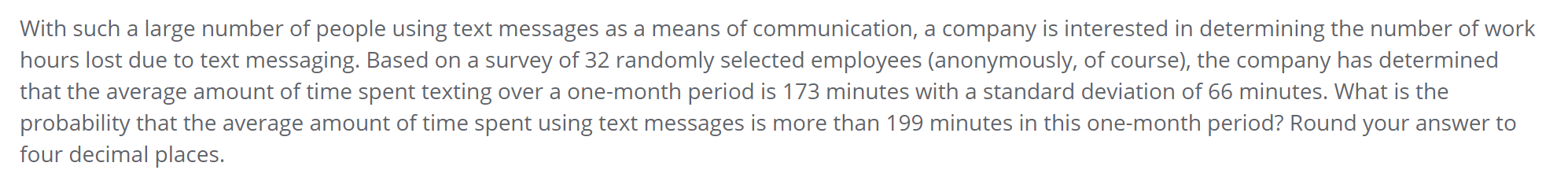# Question With such a large number of people using text messages as a means of communication, a company is interested in determining the number of work hours lost due to text messaging. Based on a survey of 32 randomly selected employees (anonymously, of course), the company has determined that the average amount of time spent texting over a one-month period is 173 minutes with a standard deviation of 66 minutes. What is the probability that the average amount of time spent using text messages is more than 199 minutes in this one-month period? Round your answer to four decimal places.JEV1IX The Asker · Probability and StatisticsTranscribed Image Text: With such a large number of people using text messages as a means of communication, a company is interested in determining the number of work hours lost due to text messaging. Based on a survey of 32 randomly selected employees (anonymously, of course), the company has determined that the average amount of time spent texting over a one-month period is 173 minutes with a standard deviation of 66 minutes. What is the probability that the average amount of time spent using text messages is more than 199 minutes in this one-month period? Round your answer to four decimal places.
More
Transcribed Image Text: With such a large number of people using text messages as a means of communication, a company is interested in determining the number of work hours lost due to text messaging. Based on a survey of 32 randomly selected employees (anonymously, of course), the company has determined that the average amount of time spent texting over a one-month period is 173 minutes with a standard deviation of 66 minutes. What is the probability that the average amount of time spent using text messages is more than 199 minutes in this one-month period? Round your answer to four decimal places.